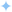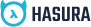Edit on GitHub

# Run mutation, update cache

Now let's do the integration part.

Firstly, we need to handle the state of the form's input element. We will use React's useReducer hook for that. Add the following code to `src/todo/TodoInput.re`.

```+type state = {+  text: string+};
+type action = HandleTextChange(string);
[@react.component]let make = (~isPublic: bool) => {+  let (state, dispatch) = React.useReducer((state, action) => {+    let HandleTextChange(text) = action;+    { text: text }+  }, { text: "" });  <form className="formInput">    <input      className="input"      placeholder="What needs to be done?"+      value=state.text+      onChange={+        event => {+          dispatch(HandleTextChange(ReactEvent.Form.target(event)##value));+        }+      }    />    <i className="inputMarker fa fa-angle-right" />  </form>}```

Now, we have the text value of the input element in the state variable called text. Next, lets pass this text value from state and the `isPublic` value from the props as query variables to our Mutation.

## Passing query variables

To add query variables to the mutation, lets use the `make` method exposed by the query module `InsertMyTodo` that we defined in the previous section. Just add the following code before returning the JSX.

`let insertTodoMutation = GraphQLQueries.InsertMyTodo.make(~todo=state.text, ~isPublic=isPublic, ());`

Now you can access the variables by using `variables` field in the `insertTodoMutation` object like `insertTodoMutation##variables`.

## Firing the mutation

Let us wrap our JSX with the `InsertMyTodoMutation` component that we defined in the last section. This component provides the `mutate` function as an argument to its render prop function. We can use this `mutate` function in the `onSubmit` handler of our form.

```type state = {  text: string};
type action = HandleTextChange(string);
[@react.component]let make = (~isPublic: bool) => {  let (state, dispatch) = React.useReducer((_, action) => {    let HandleTextChange(text) = action;    { text: text }  }, { text: "" });+  let insertTodoMutation = GraphQLQueries.InsertMyTodo.make(~todo=state.text, ~isPublic=isPublic, ());+  <GraphQLQueries.InsertMyTodoMutation>+    ...{+      (mutate, _) => {        <form className="formInput">          <input            className="input"            placeholder="What needs to be done?"            value=state.text            onChange={              event => {                dispatch(HandleTextChange(ReactEvent.Form.target(event)##value));              }            }          />          <i className="inputMarker fa fa-angle-right" />        </form>+      }+    }+  </GraphQLQueries.InsertMyTodoMutation>};```

Now that we have the `mutate` function in our scope, let us call this function in the `onSubmit` handler of the `form`.

`-<form className="formInput">+<form className="formInput" onSubmit={+  event => {+     ReactEvent.Form.preventDefault(event);+     mutate(+       ~variables=insertTodoMutation##variables,+       ()+     ) |> ignore;+  }+}>`

After this, if you type a todo and press enter, you can see the mutation in the network request being sent in your browser's dev tools. Look at the `mutate` function closely. We are calling it by passing the variables with an optional labelled argument called `~variables`.

But the UI does not get updated unless you refresh the page. To update the UI after every mutation, we want to use another optional argument of the `mutate` function called `refetchQueries`. Lets use it as follows:

```
mutate(  ~variables=insertTodoMutation##variables,+ ~refetchQueries=[|"getMyTodos"|],  ()) |> ignore;```

`refetchQueries` helps in keeping the local state and the server state in sync. It takes an array of query names and fetches syncs the data accordingly. In our case, we passed a query with operation name called `getMyTodos` because that's the data we wish to sync. Now if you try typing something and pressing enter, now you would see the UI being updated.

Lastly, you want to clear the textbox if the mutation is successful. To achieve that, add the following code to the form's event handler:

`event => {   ReactEvent.Form.preventDefault(event);   mutate(     ~variables=insertTodoMutation##variables,     ~refetchQueries=[|"getMyTodos"|],     ()   )+   |> Js.Promise.then_(data => {+      dispatch(HandleTextChange(""));+      Js.Promise.resolve()+   })   |> ignore;}`

The `mutate` function returns a promise. We are clearing off the textbox when the promise gets resolved i.e. when the mutation is successful.

Awesome! You have used your first Mutation component to fire a mutation.

•Build apps and APIs 10x faster
•Built-in authorization and caching
•8x more performant than hand-rolled APIs